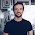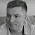## Tuesday, October 20, 2015

### Brain teaser

Friend of mine ask me a question: how to switch values of two variables (Integer type to be strict) but without using another one?

Use this spock spec as problem definition:
 @Unroll def "should switch variables (#a,#b) without additional variable"() { expect: new Switcher(a, b).switchVariables().toString() == result where: a | b | result 1 | 2 | "a=2 b=1" 10 | 20 | "a=20 b=10" -10 | -20 | "a=-20 b=-10" 20 | 10 | "a=10 b=20" -20 | -10 | "a=-10 b=-20" 0 | 10 | "a=10 b=0" 10 | 0 | "a=0 b=10" -10 | 0 | "a=0 b=-10" 0 | 0 | "a=0 b=0" 0 | -1 | "a=-1 b=0" 10 | 10 | "a=10 b=10" Integer.MAX_VALUE | Integer.MAX_VALUE | "a=" + Integer.MAX_VALUE + " b=" + Integer.MAX_VALUE Integer.MIN_VALUE | Integer.MAX_VALUE | "a=" + Integer.MAX_VALUE + " b=" + Integer.MIN_VALUE Integer.MIN_VALUE | Integer.MIN_VALUE | "a=" + Integer.MIN_VALUE + " b=" + Integer.MIN_VALUE 1 | Integer.MAX_VALUE | "a=" + Integer.MAX_VALUE + " b=1" Integer.MIN_VALUE | 1 | "a=1 b=" + Integer.MIN_VALUE -1 | Integer.MIN_VALUE | "a=" + Integer.MIN_VALUE + " b=-1" }
You can find working solution on https://github.com/piotrpietrzak/gatchaman/tree/swicher
Have fun!

#### 2 comments:

1.There is this handy XCHG assembly instruction for that ;-)
http://x86.renejeschke.de/html/file_module_x86_id_328.html

1.On 6502 assembly you can do "almost" the same with x and y registers:
setup lda #1
sta \$7e
lda #2
sta \$7f

switch ldx \$7e
ldy \$7f
stx \$7f
sty \$7e
Those ldx,ldy,stx,sty instrustions will take only 3 cycles (12 cycles overall) to complete and this ugly XCHG >15 but I can't find XCHG mem, mem and therefore you need to do XCHG reg,mem and load and store will cost you extra cycles.

I found someting on paper you decided to cite:
Temporary = Destination;
Destination = Source;
Source = Temporary;
This is not the proper solution of this puzzle:)